Structural Bioinformatics Library Template C++ / Python API for developping structural bioinformatics applications.
User Manual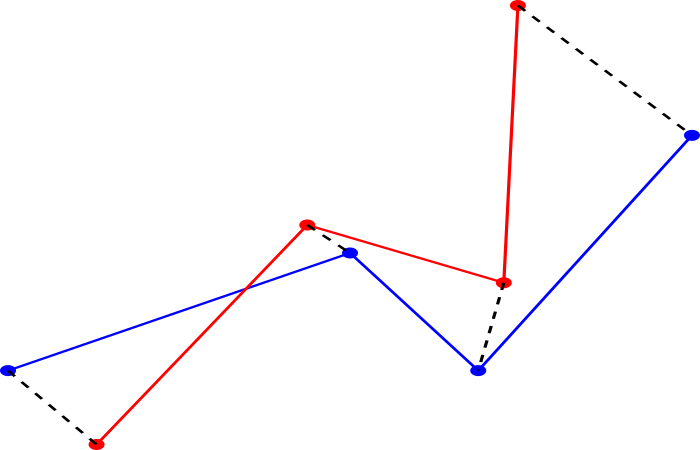# Point_cloud_rigid_registration_3

Authors: F. Cazals and T. Dreyfus

# Introduction

Consider two point sets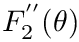and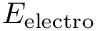of the same size; we also assume an alignment between them, meaning that the i-th point ofis paired with the i-th point from.

The point set registration consists on finding a spatial transformation aligningonto. The registration is said rigid if the distance between all pairs of points is conserved by the transformation. This package defines a rigid registration between two set of 3D points where the spatial transformation is a composition of translations and rotations.

# Implementation

## Theory

Assume that we want to perform the registration of the point cloudonto the point cloud. In doing so, we shall assume that the center of mass (aka centroid) ofis the reference center of mass, and upon applying the optimal rigid motion to, the centroid of this modified point set will coincide with that of.

The rigid registration is done using a linear algebra algorithm that computes the optimal rotation matrix between the two input set of points using the Singular Value Decomposition (SVD) of their covariance matrix. Basically, once centered on the origin, the covariance matrix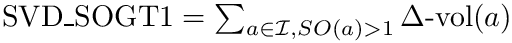of the two input sets of pointsandis computed. The SVD of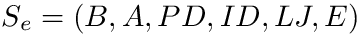then produces orthogonal matrices representing rotations (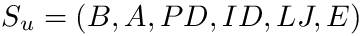).

More precisely, the algorithm is as follows :

• 1) compute the centroids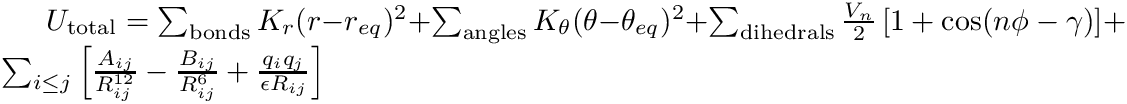and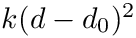ofandrespectively, so as to center the input set of points, and fills the matrices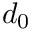and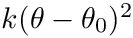of size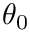and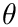with the new centered points;
• 2) compute the covariance matrix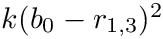;
• 3) compute the SVD of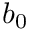;
• 4) compute the matrix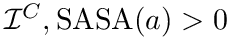that is the identity matrix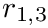, except that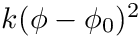, and then computes the optimal rotation matrix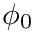;
• 5) rotate the matrixusing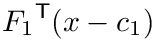; denoting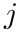the origin of the coordinate system, further translate the resulting point cloud with the vector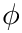for its center of mass to coincide with that of.

## Design

The algorithm is coded in the class SBL::GT::T_Point_cloud_rigid_registration_3< FT >, where FT is the number type used for representing the coordinates of the points (by default, the double type).

The class can be used in two modes :

• 1) using two point clouds and computing directly the associated rigid registration;
• 2) using two point clouds to initialize a transformation that can be reused anytime.

Note that the second one is suited to cases where the same transformation has to be applied to a family of point clouds.

The constructor of SBL::GT::T_Point_cloud_rigid_registration_3 takes two containers of points and computes the associated rigid transformation. Then the class provides functors and methods for the aforementioned two modes :

• 1) Functor taking two point clouds as argument: computes and stores internally the transformation defined by these two point clouds.
• 2) Method transform taking one point cloud as argument: uses the internal transformation to transform this point cloud.

For both modes, the input points clouds can be represented in three different ways :

• random-access containers of points (e.g arrays or vectors),
• pairs of (begin, end) iterators over the containers of points,
• triples (size, begin, end), corresponding to the size and iterators over containers of the coordinates of the input points.

The last one is useful when the input sets of points are represented by D-dimensional points, for exemple when computing the distance between molecular conformations (see package Molecular_distances)

# Examples

## Registration with rotated and translated set of points

The following example shows how to perform a rigid registration:

• A point set is loaded (variable points),
• This point set is rotated (variable points_transformed),
• points_transformed in then registered onto points (variable points_registered).
• It is then checked that points and points_registered coincide (see also the l-RMSD calculation in package Molecular_distances).
#include <iostream>
#include <fstream>
#include <random>
#include <SBL/GT/Point_cloud_rigid_registration_3.hpp>
#include <CGAL/Cartesian.h>
typedef CGAL::Cartesian<double> K;
typedef K::Point_3 Point_3;
int main(int argc, char *argv[])
{
//Read 3D points from an input file
if(argc < 2) return -1;
std::vector<Point_3> points;
std::ifstream in(argv);
while(!in.eof())
{
Point_3 p; in >> p;
points.push_back(p);
}
in.close();
std::vector<K::Point_3> points_registered;
std::vector<Point_3> points_transformed;
std::mt19937 gen(1); //Standard mersenne_twister_engine seeded with rd()
std::uniform_real_distribution<> dis(0.0, 1.0);
//Rotates and translates the input points
for(unsigned i = 0; i < points.size(); i++)
{
K::FT x = std::cos(CGAL_PI/4)*points[i].x() - std::sin(CGAL_PI/4)*points[i].y();
K::FT y = std::sin(CGAL_PI/4)*points[i].x() + std::cos(CGAL_PI/4)*points[i].y();
K::FT noize = dis(gen);
points_transformed.push_back(Point_3(x+20, y+noize, points[i].z()));
}
//Makes the registration
registration(points, points_transformed, std::back_inserter(points_registered));
//Comptes the distance between pairs of 3D points
K::FT d = 0;
for(unsigned i = 0;i < points.size(); i++)
d += (points[i] - points_registered[i]).squared_length()/(K::FT)points.size();
std::cout << "Rotated and Translated Registration: " << d << std::endl;
return 0;
}
Model of Distances for defining distance between two point clouds.
Definition: Point_cloud_rigid_registration_3.hpp:115
d
Definition: extract-wales-graph.py:73
p
Definition: extract-wales-graph.py:74
z
Definition: generate-random-balls-3.py:26
y
Definition: generate-random-balls-3.py:25
x
Definition: generate-random-balls-3.py:24

## Multiple rigid registrations

The following example shows how to store the transformation defined by the rigid registration of a point cloud onto another one, so as to later use this transformation to transform any point cloud.

Note that in this example, variable points2 is registered onto points1, so that the reference centroid is that of point1. Which means that in applying the optimal rigid motion to points3, the centroid of the transformed points3 coincides with that of points1.

#include <iostream>
#include <fstream>
#include <random>
#include <SBL/GT/Point_cloud_rigid_registration_3.hpp>
#include <CGAL/Cartesian.h>
typedef CGAL::Cartesian<double> K;
typedef K::Point_3 Point_3;
int main(int argc, char *argv[])
{
//Read 3D points from an input file
if(argc < 4) return -1;
std::vector<Point_3> points1;
std::vector<Point_3> points2;
std::vector<Point_3> points3;
std::ifstream in1(argv);
while(!in1.eof())
{
Point_3 p; in1 >> p;
points1.push_back(p);
}
in1.close();
std::ifstream in2(argv);
while(!in2.eof())
{
Point_3 p; in2 >> p;
points2.push_back(p);
}
in2.close();
std::ifstream in3(argv);
while(!in3.eof())
{
Point_3 p; in3 >> p;
points3.push_back(p);
}
in3.close();
Point_cloud_rigid_registration_3 registration(points1, points2);
std::vector<Point_3> points_transformed;
registration.transform(points3, std::back_inserter(points_transformed));
//Print the transformed points
K::FT d = 0;
for(unsigned i = 0;i < points1.size(); i++)
{
std::cout << points_transformed[i] << std::endl;
d += (points1[i] - points_transformed[i]).squared_length();
}
std::cout << "Distance from first set of points and the transformed third set of points : " << d << std::endl;
std::cout << "Reference centroid : " << registration.get_reference_centroid() << std::endl;
return 0;
}
const Point_3 & get_reference_centroid(void) const
Return the reference centroid if any.
Definition: Point_cloud_rigid_registration_3.hpp:609
OutputIterator transform(const PointContainer &points, OutputIterator out) const
Make the rigid registration of the input points w.r.t the internal transformation,...
Definition: Point_cloud_rigid_registration_3.hpp:617

# Applications

This package offers alors a program for performing the rigid registration between a reference set of 3D points and a collection of sets of 3D points : sbl-align-point-clouds-3.exe . An input set of 3D points is represented as a D dimensional point, where D is divisable by 3. A file listing D dimensional points is a text file where each point is represented by its dimension followed by its coordinates. Note that breaklines are not considered, so that having one D dimensional point per line, or one 3D point per line are both acceptable :

```6 0 0 0 1 1 1
```

or

```6
0 0 0
1 1 1
```

The program sbl-align-point-clouds-3.exe takes two files as input : the first one containing one D dimensional point representing the reference set of points, and the second containing any number of D dimensional points.